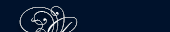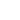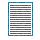НОВОСТИ   БИБЛИОТЕКА   ЭНЦИКЛОПЕДИЯ   КАРТА САЙТА   ССЫЛКИ   О САЙТЕ### THE PROCESS ОF SUBTRACTION

Subtraction of many-place numbers is performed as follows:We begin the subtraction from the right and we subtract the numbers in the same column. Thus: 6-4 = 2; 8 - 5=3; etc.

#### Multiplication of Whole Numbers

Multiplication is an arithmetic operation by means of which one number is repeated as an addend until it occurs as many times as it is indicated by another number.

There are two numbers involved in multiplication. The result of their operation on each other is known as the product.

That number which is repeated as an addend is known as the multiplicand. The number by which the multiplicand is multiplied is known as the multiplier. The sign is × or (•).

#### Division of Whole Numbers

The operation by means of which a factor is obtained when the product and the other factor are given is called division.

The arithmetic operation of division is performed on that number which we take as the given product.

In division this number is called the dividend.

The given factor is known as the number by which the dividend or product is to be divided. This number is called the divisor.

The result of the division of the dividend by the divisor is called the quotient. The sign of division is : or ( ÷in England).ПОИСК: